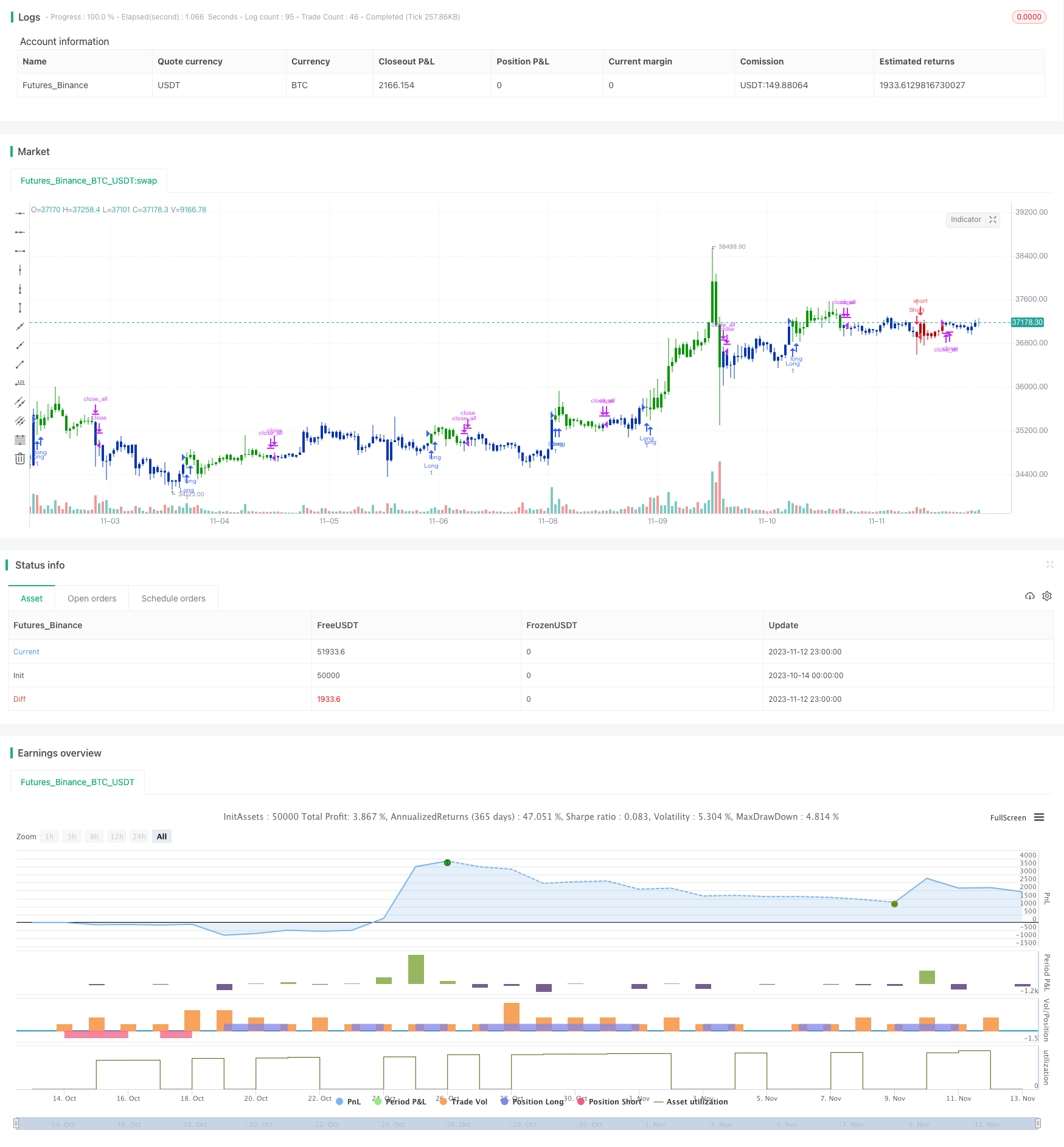Author: ChaoZhang, Date: 2023-11-14 13:42:47
Tags:## Overview

The dual-confirmation reversal trading strategy combines the 123 reversal pattern with the Stochastic RSI indicator to create a robust mean-reversion system. It provides two layers of confirmation before entering a trade, improving the strategy’s accuracy and stability.

## Strategy Logic

The strategy consists of two components:

1. 123 Reversal

It uses the 123 pattern to identify potential reversals. The logic is:

• Long if close < previous close and current close > previous close and 9-day Slow Stochastic < 50

• Short if close > previous close and current close < previous close and 9-day Fast Stochastic > 50

This provides an early signal for price reversals.

1. Stochastic RSI

It applies Stochastic indicator on RSI for additional confirmation:

• Compute RSI with length 14

• Calculate Stochastic of RSI, with lengths 14, to get K

• Take 3-day SMA of K to get D

• If K crosses above 80, it indicates long. If K crosses below 20, it indicates short.

A trade is triggered only when both parts agree.

The key advantage of this strategy is the double confirmation, which improves accuracy and reduces whipsaws. Specific benefits include:

1. 123 reversal provides early detection of trend reversal

2. Stochastic RSI confirms the reversal signal

3. Combination improves win rate and reduces false signals

4. Parameters can be optimized for different markets

5. Simple and clean implementation for live trading

## Risk Analysis

Some risks to consider for this strategy:

1. Failed reversal risk. False reversals may cause losses.

3. Overfitting risk. Excessive optimization to historical data.

4. High trading frequency risk. More signals may increase costs.

5. Coding error risk. Bugs in implementation logic.

Possible solutions:

1. Use prudent position sizing to limit losses.

2. Employ walk-forward optimization methods.

3. Focus on parameter stability, not high returns.

4. Tune conditions to reduce trade frequency.

5. Thoroughly test code logic.

## Enhancement Opportunities

The strategy can be improved in the following areas:

1. Parameter tuning for specific markets.

2. Adding filters to avoid hasty reversals.

3. Incorporating stop loss mechanisms.

5. Implementing dynamic position sizing.

## Conclusion

The dual-confirmation reversal strategy is a stable and practical system for short-term mean-reversion. It balances the sensitivity to catch reversals and the accuracy from dual confirmation. With proper optimization and modifications, it can effectively complement a quantitative strategy portfolio. But parameters should be robust and risks like overfitting and whipsaws should be managed prudently in live trading.

```/*backtest
start: 2023-10-14 00:00:00
end: 2023-11-13 00:00:00
period: 1h
basePeriod: 15m
exchanges: [{"eid":"Futures_Binance","currency":"BTC_USDT"}]
*/

//@version=4
////////////////////////////////////////////////////////////
//  Copyright by HPotter v1.0 03/08/2021
// This is combo strategies for get a cumulative signal.
//
// First strategy
// This System was created from the Book "How I Tripled My Money In The
// Futures Market" by Ulf Jensen, Page 183. This is reverse type of strategies.
// The strategy buys at market, if close price is higher than the previous close
// during 2 days and the meaning of 9-days Stochastic Slow Oscillator is lower than 50.
// The strategy sells at market, if close price is lower than the previous close price
// during 2 days and the meaning of 9-days Stochastic Fast Oscillator is higher than 50.
//
// Second strategy
// This strategy used to calculate the Stochastic RSI
//
// WARNING:
// - For purpose educate only
// - This script to change bars colors.
////////////////////////////////////////////////////////////
Reversal123(Length, KSmoothing, DLength, Level) =>
vFast = sma(stoch(close, high, low, Length), KSmoothing)
vSlow = sma(vFast, DLength)
pos = 0.0
pos := iff(close < close and close > close and vFast < vSlow and vFast > Level, 1,
iff(close > close and close < close and vFast > vSlow and vFast < Level, -1, nz(pos, 0)))
pos

SRSI(lengthRSI,lengthStoch,smoothK,smoothD, TopBand,LowBand) =>
pos = 0.0
Source = close
rsi1 = rsi(Source, lengthRSI)
k = sma(stoch(rsi1, rsi1, rsi1, lengthStoch), smoothK)
d = sma(k, smoothD)
d_cross_80 = cross(d,TopBand)
pos := iff(k > TopBand, 1,
iff(k < LowBand, -1, nz(pos, 0)))
pos

strategy(title="Combo Backtest 123 Reversal & Stochastic RSI", shorttitle="Combo", overlay = true)
line1 = input(true, "---- 123 Reversal ----")
Length = input(14, minval=1)
KSmoothing = input(1, minval=1)
DLength = input(3, minval=1)
Level = input(50, minval=1)
//-------------------------
line2 = input(true, "---- Stochastic RSI ----")
TopBand = input(80, step=0.01)
LowBand = input(20, step=0.01)
lengthRSI = input(14, minval=1)
lengthStoch = input(14, minval=1)
smoothK = input(3, minval=1)
smoothD = input(3, minval=1)
posReversal123 = Reversal123(Length, KSmoothing, DLength, Level)
posSRSI = SRSI(lengthRSI,lengthStoch,smoothK,smoothD, TopBand,LowBand)
pos = iff(posReversal123 == 1 and posSRSI == 1 , 1,
iff(posReversal123 == -1 and posSRSI == -1, -1, 0))
possig = iff(reverse and pos == 1, -1,
iff(reverse and pos == -1 , 1, pos))
if (possig == 1 )
strategy.entry("Long", strategy.long)
if (possig == -1 )
strategy.entry("Short", strategy.short)
if (possig == 0)
strategy.close_all()
barcolor(possig == -1 ? #b50404: possig == 1 ? #079605 : #0536b3 )
```

More